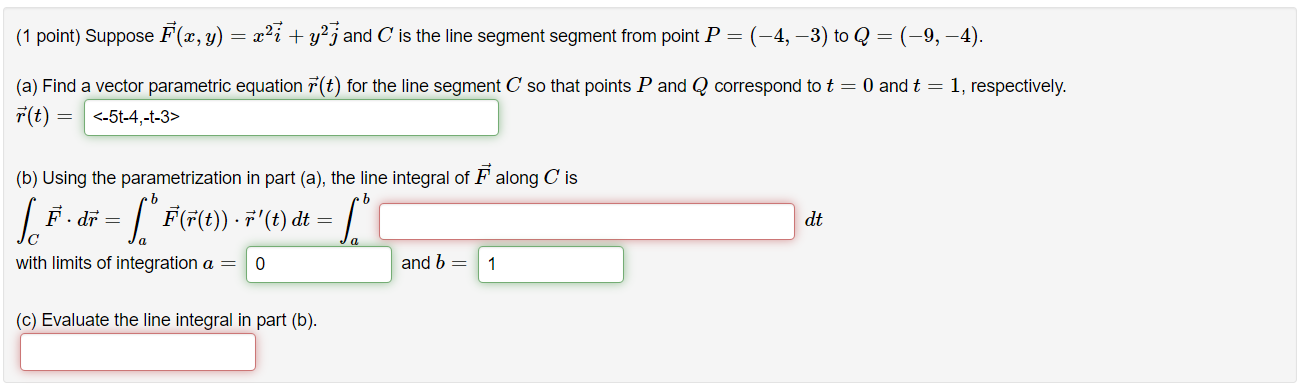### Create an Account

Already have account?

### Forgot Your Password ?

Home / Questions / (1 point) Suppose F(x, y) = x27 + y2j and C is the line segment segment from point P=(-4, ...

# (1 point) Suppose F(x, y) = x27 + y2j and C is the line segment segment from point P=(-4, -3) to Q = (–9,-4). (a) Find a vector parametric equation F(t) for the line segment C so that points P and Q c

(1 point) Suppose F(x, y) = x27 + y2j and C is the line segment segment from point P=(-4, -3) to Q = (–9,-4). (a) Find a vector parametric equation F(t) for the line segment C so that points P and Q correspond to t = 0 and t = 1, respectively. r(t) = (b) Using the parametrization in part (a), the line integral along C is lo F. dr = | * F(r(e) . '(t) dt dt a with limits of integration a = 0 and b= 1 (c) Evaluate the line integral in part (b).Apr 14 2021 View more View Less

#### Answer (Solved)Subscribe To Get Solution